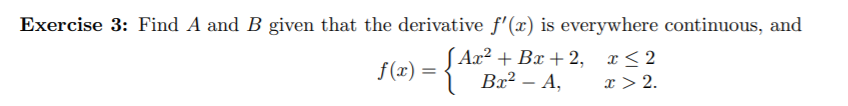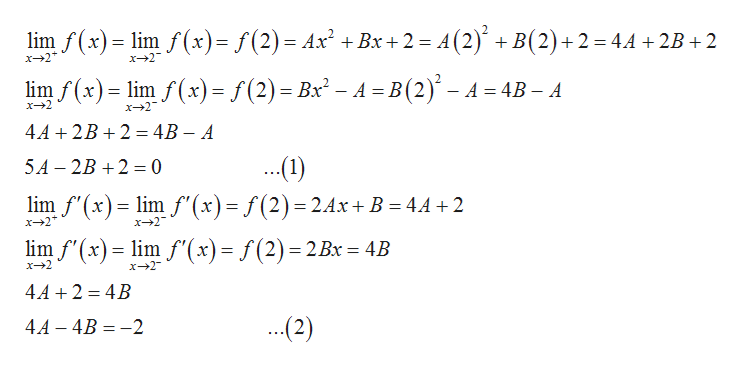# Exercise 3: Find A and B given that the derivative f'(r) is everywhere continuous, andAa2 Bx 2, x

Questionhelp_outlineImage TranscriptioncloseExercise 3: Find A and B given that the derivative f'(r) is everywhere continuous, and Aa2 Bx 2, x<2 Br2-A f(a) x 2 fullscreen
check_circle

Step 1

The function f(x) whose derivative is everywhere continuous is given by,

Step 2

The values of A and B are comput...help_outlineImage Transcriptioncloselim f(x)lim f (x)= f(2) = Ax2 +Bx 2 = A(2) B(2)+2 4.4 2B 2 x 2 x 2 /(x)= lim f(x)= f(2) = Bx2 - A =B(2)- A- В 4B - A x+2 x 2 4A+2B 24B - A (1) lim f'(x) im f" (x) = f(2) = 2.4x+ B = 44+2 5A 2B 2 0 x--2 x 2 lim f'(x)lm f'(x)= f(2) 2Bx = 4B x 2 x2 4A 2 4B (2) 4A -4B 2 fullscreen

### Want to see the full answer?

See Solution

#### Want to see this answer and more?

Solutions are written by subject experts who are available 24/7. Questions are typically answered within 1 hour.*

See Solution
*Response times may vary by subject and question.
Tagged in

### Functions